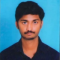## Solve each of the following equations and also check your results in each case:(i) $\frac{45-2x}{15}-\frac{4x+10}{5}=\frac{15-14x}{9}$(ii) $\frac{5(7x+5)}{3}-\frac{23}{3}=13-\frac{4x-2}{3}$Akhileshwar Nani

Updated on 13-Apr-2023 23:12:26

57 Views

Given:The given equations are:(i) $\frac{45-2x}{15}-\frac{4x+10}{5}=\frac{15-14x}{9}$(ii) $\frac{5(7x+5)}{3}-\frac{23}{3}=13-\frac{4x-2}{3}$To do:We have to solve the given equations and check the results.Solution:To check the results we have to find the values of the variables and substitute them in the equation. Find the value of LHS and the value of RHS and check whether both are ... Read More

## Solve each of the following equations and also check your results in each case:(i) $\frac{2}{3x}-\frac{3}{2x}=\frac{1}{12}$(ii) $\frac{4x}{9}+\frac{1}{3}+\frac{13x}{108}=\frac{8x+19}{18}$Akhileshwar Nani

Updated on 13-Apr-2023 23:11:29

53 Views

Given:The given equations are:(i) $\frac{2}{3x}-\frac{3}{2x}=\frac{1}{12}$(ii) $\frac{4x}{9}+\frac{1}{3}+\frac{13x}{108}=\frac{8x+19}{18}$To do:We have to solve the given equations and check the results.Solution:To check the results we have to find the values of the variables and substitute them in the equation. Find the value of LHS and the value of RHS and check whether both are ... Read More

## Solve each of the following equations and also check your results in each case:(i) $\frac{9x+7}{2}-(x-\frac{(x-2)}{7})=36$(ii) $0.18(5x-4)=0.5x+0.8$Akhileshwar Nani

Updated on 13-Apr-2023 23:10:16

68 Views

Given:The given equations are:(i) $\frac{9x+7}{2}-(x-\frac{(x-2)}{7})=36$(ii) $0.18(5x-4)=0.5x+0.8$To do:We have to solve the given equations and check the results.Solution:To check the results we have to find the values of the variables and substitute them in the equation. Find the value of LHS and the value of RHS and check whether both are ... Read More

## Solve each of the following equations and also check your results in each case:(i) $\frac{3x+1}{16}+\frac{2x-3}{7}=\frac{x+3}{8}+\frac{3x-1}{14}$(ii) $\frac{1-2x}{7}-\frac{2-3x}{8}=\frac{3}{2}+\frac{x}{4}$Akhileshwar Nani

Updated on 13-Apr-2023 23:09:37

47 Views

Given:The given equations are:(i) $\frac{3x+1}{16}+\frac{2x-3}{7}=\frac{x+3}{8}+\frac{3x-1}{14}$(ii) $\frac{1-2x}{7}-\frac{2-3x}{8}=\frac{3}{2}+\frac{x}{4}$To do:We have to solve the given equations and check the results.Solution:To check the results we have to find the values of the variables and substitute them in the equation. Find the value of LHS and the value of RHS and check whether both are ... Read More

## Solve each of the following equations and also check your results in each case:(i) $\frac{3x}{4}-\frac{x-1}{2}=\frac{x-2}{3}$(ii) $\frac{5x}{3}-\frac{(x-1)}{4}=\frac{(x-3)}{5}$Akhileshwar Nani

Updated on 13-Apr-2023 23:08:58

69 Views

Given:The given equations are:(i) $\frac{3x}{4}-\frac{x-1}{2}=\frac{x-2}{3}$(ii) $\frac{5x}{3}-\frac{(x-1)}{4}=\frac{(x-3)}{5}$To do:We have to solve the given equations and check the results.Solution:To check the results we have to find the values of the variables and substitute them in the equation. Find the value of LHS and the value of RHS and check whether both are ... Read More

## Solve each of the following equations and also check your results in each case:(i) $\frac{(3a-2)}{3}+\frac{(2a+3)}{2}=a+\frac{7}{6}$(ii) $x-\frac{(x-1)}{2}=1-\frac{(x-2)}{3}$Akhileshwar Nani

Updated on 13-Apr-2023 23:08:12

63 Views

Given:The given equations are:(i) $\frac{(3a-2)}{3}+\frac{(2a+3)}{2}=a+\frac{7}{6}$(ii) $x-\frac{(x-1)}{2}=1-\frac{(x-2)}{3}$To do:We have to solve the given equations and check the results.Solution:To check the results we have to find the values of the variables and substitute them in the equation. Find the value of LHS and the value of RHS and check whether both are ... Read More

## Solve each of the following equations and also check your results in each case:(i) $\frac{7x}{2}-\frac{5x}{2}=\frac{20x}{3}+10$(ii) $\frac{6x+1}{2}+1=\frac{7x-3}{3}$Akhileshwar Nani

Updated on 13-Apr-2023 23:07:25

43 Views

Given:The given equations are:(i) $\frac{7x}{2}-\frac{5x}{2}=\frac{20x}{3}+10$(ii) $\frac{6x+1}{2}+1=\frac{7x-3}{3}$To do:We have to solve the given equations and check the results.Solution:To check the results we have to find the values of the variables and substitute them in the equation. Find the value of LHS and the value of RHS and check whether both are ... Read More

## Solve each of the following equations and also check your results in each case:(i) $\frac{1}{2}x+7x-6=7x+\frac{1}{4}$(ii) $\frac{3}{4}x+4x=\frac{7}{8}+6x-6$Akhileshwar Nani

Updated on 13-Apr-2023 23:06:27

51 Views

Given:The given equations are:(i) $\frac{1}{2}x+7x-6=7x+\frac{1}{4}$(ii) $\frac{3}{4}x+4x=\frac{7}{8}+6x-6$To do:We have to solve the given equations and check the results.Solution:To check the results we have to find the values of the variables and substitute them in the equation. Find the value of LHS and the value of RHS and check whether both are ... Read More

## Solve each of the following equations and also check your results in each case:(i) $\frac{7y+2}{5}=\frac{6y-5}{11}$(ii) $x-2x+2-\frac{16}{3}x+5=3-\frac{7}{2}x$Akhileshwar Nani

Updated on 13-Apr-2023 23:05:12

48 Views

Given:The given equations are:(i) $\frac{7y+2}{5}=\frac{6y-5}{11}$(ii) $x-2x+2-\frac{16}{3}x+5=3-\frac{7}{2}x$To do:We have to solve the given equations and check the results.Solution:To check the results we have to find the values of the variables and substitute them in the equation. Find the value of LHS and the value of RHS and check whether both are ... Read More

## Solve each of the following equations and also check your results in each case:(i) $\frac{2x+5}{3}=3x-10$(ii) $\frac{a-8}{3}=\frac{a-3}{2}$Akhileshwar Nani

Updated on 13-Apr-2023 23:03:34

46 Views

Given:The given equations are:(i) $\frac{2x+5}{3}=3x-10$(ii) $\frac{a-8}{3}=\frac{a-3}{2}$To do:We have to solve the given equations and check the results.Solution:To check the results we have to find the values of the variables and substitute them in the equation. Find the value of LHS and the value of RHS and check whether both are ... Read More